Courses

# Test: Fluid Machines - 3

## 20 Questions MCQ Test Topicwise Question Bank for Civil Engineering | Test: Fluid Machines - 3

Description
This mock test of Test: Fluid Machines - 3 for Civil Engineering (CE) helps you for every Civil Engineering (CE) entrance exam. This contains 20 Multiple Choice Questions for Civil Engineering (CE) Test: Fluid Machines - 3 (mcq) to study with solutions a complete question bank. The solved questions answers in this Test: Fluid Machines - 3 quiz give you a good mix of easy questions and tough questions. Civil Engineering (CE) students definitely take this Test: Fluid Machines - 3 exercise for a better result in the exam. You can find other Test: Fluid Machines - 3 extra questions, long questions & short questions for Civil Engineering (CE) on EduRev as well by searching above.
QUESTION: 1

Solution:
QUESTION: 2

### The cavitation and pitting can be prevented by creating which one of the following conditions? .

Solution:

Cavitation occurs due to pressure falling below vapour pressure. Reducing the velocity head will increase pressure head and cavitation can be prevented.

QUESTION: 3

### The overall efficiency of a Pelton turbine is 70%. If the mechanical efficiency is 85%, what is its hydraulic efficiency?

Solution:

η0 = ηn x ηm
∴  hydraulic eff. = (0.70/0.85) x 100 = 82.4%

QUESTION: 4

The function of an air vessel in a reciprocating pump is to obtain :

Solution:

Air vessel ensure continuous supply of water at a uniform rate in a reciprocating pump. It results in saving of work against friction.
Percentage of work saved during stroke in
(i) single acting pump is 84.8%
(ii) double acting pump is 39.2%
The air vessels change the indicator diagram of the reciprocating pump.

QUESTION: 5

A centrifugal pump gives maximum efficiency when its impeller blades are

Solution:
QUESTION: 6

The speed factor of a Peiton turbine for maximum efficiency condition is about

Solution:

Speed factor: The ratio of the bucket speed uand the theoretical velocity of jet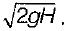QUESTION: 7

The specific speed of a pump of discharge Q, working under a head H at an RPM ‘n' is NSP =

Solution:
QUESTION: 8

If two pumps identical in all aspects and each capable of delivering a discharge Q against a head H are connected

Solution:
QUESTION: 9

Cavitatio  parameter σ is defined in terms of NPSH (net. positive suction head) and net head H as, σ=

Solution:
QUESTION: 10

Cavitation in fluid flow occurs when

Solution:
QUESTION: 11

The minimum head a cross the impeller for the delivery to commence is

Solution:
QUESTION: 12

Rotodyn a micpumps a reclassified as
1. centrifugal pumps
2. screw pumps
3. propeller pumps
Which of these statements are correct?

Solution:
QUESTION: 13

For lifting oil from very deep oil wells, which of the pumps are used?

Solution:
QUESTION: 14

The efficiency of a centrifugal pump is expressed in terms of
1. manometer efficiency
2. volumetric efficiency
3. mechanical efficiency
4. overall efficiency
Which of these statements are correct?

Solution:
QUESTION: 15

A two-dimensional jet strikes a fixed two- dimensional plane at 45° to the normal to the plane, This causes the jet to split into two streams whose discharges are in the ratio

Solution: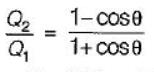Q = 180° - 45°

QUESTION: 16

To generate 10,000 HP under a head of 81 m while working at a speed of 500 rpm, the turbine of choice would be

Solution:

Power developed in kW
= 10,000 x 0.756 = 7560 kW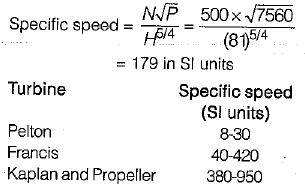QUESTION: 17

Owing to a sudden closure of turbines in a powerhouse:

Solution:

If the sluice gate of the head race channel is suddenly closed (for closing turbine) positive surge will move upstream. If the sluice gate is suddenly opened (to start turbine) positive surge will move downstream.

QUESTION: 18

A centrifugal pump running at 500 rpm and at its maximum efficiency is delivering a head of 30 m at a flow rate of 60 liters per minute. If the rpm is changed to 1000, then the head H in metres and flow rate Q in litres per minute at maximum efficiency are estimated to be

Solution: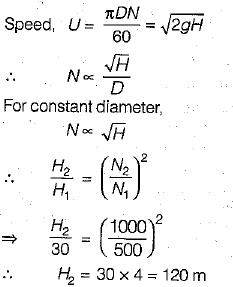We also know that specific speed will be constant.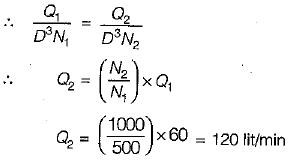QUESTION: 19

Tests were conducted on a francis turbine of 0.8 m diameter under a head of 9m. The turbine running at 240 rpm develops 84.5 kW and the water consumption was 1.2 m3/s. If the some turbine operated under a head of 16 m, the new discharge will be

Solution: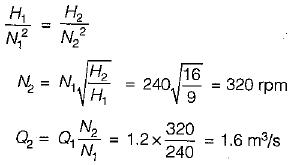QUESTION: 20

The overall efficiency of a turbine is dependent on the hydraulic loss, volumetric losses and mechanical losses represent by respective efficiencies. It is related to these by the following relationship

Solution: